libxlsxwriter
Working with Worksheet Tables

Tables in Excel are a way of grouping a range of cells into a single entity that has common formatting or that can be referenced from formulas. Tables can have column headers, autofilters, total rows, column formulas and default formatting.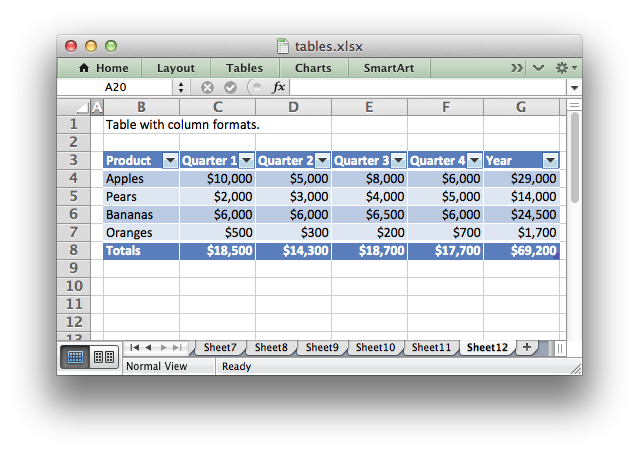For a general introduction to this Excel feature see An Overview of Excel Tables in the Microsoft Office documentation.

# Adding a table to a worksheet

Tables are added to a worksheet using the `worksheet_add_table()` function:

worksheet_add_table(worksheet, 2, 1, 6, 5, NULL);

Or more explicitly using the RANGE() macro:

worksheet_add_table(worksheet, RANGE("B3:F7"), NULL); //Same as above.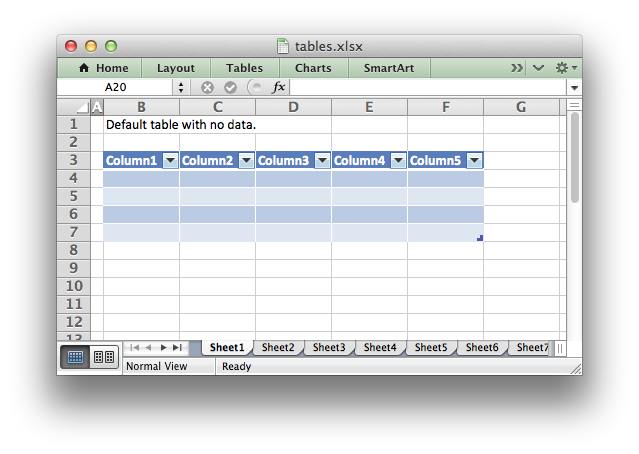The `worksheet_add_table()` `options` parameter should be a pointer to a lxw_table_options struct with the parameters that describe the table options:

worksheet_add_table(worksheet, 2, 1, 6, 5, &options);

These options are explained in the sections below. There are no required parameters and the `options` parameter is itself optional, in which case you can specify NULL and get the default table parameters.

You should take care not to overlap worksheet tables as this is not allowed by Excel and will cause an error when the file is loaded.

Note
Tables aren't available in libxlsxwriter when using `constant_memory` mode in `workbook_new_opt()`.

The `no_header_row` parameter can be used to turn off the header row in the table. It is on by default:

lxw_table_options options = {.no_header_row = LXW_TRUE};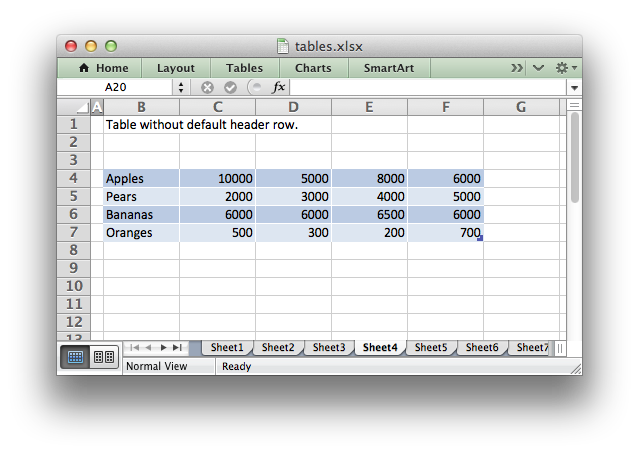Without this option the header row will contain default captions such as `Column 1`, `Column 2`, etc. These captions can be overridden using the `columns` parameter shown below.

## Parameter: no_autofilter

The `no_autofilter` parameter can be used to turn off the autofilter in the header row. It is on by default: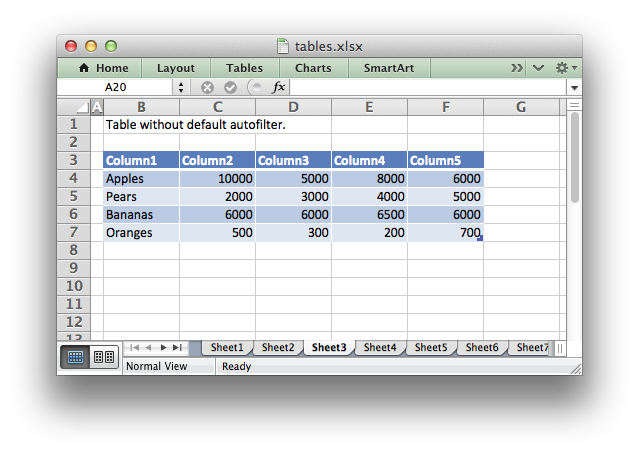The autofilter is only shown if the `no_header_row` parameter is off (the default). Filter conditions within the table are not supported.

## Parameter: no_banded_rows

The `no_banded_rows` parameter can be used to turn off the rows of alternating color in the table. It is on by default: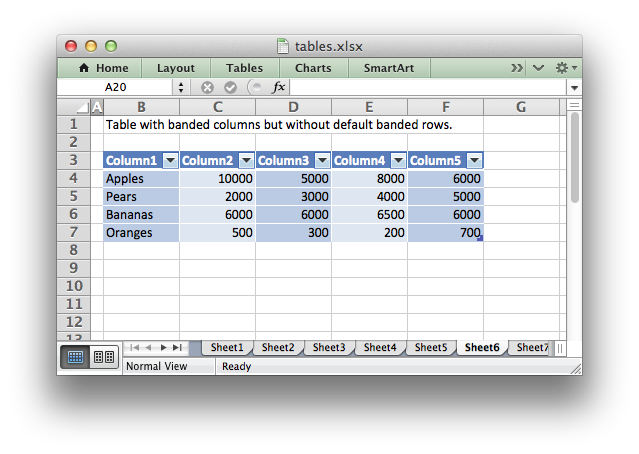## Parameter: banded_columns

The `banded_columns` parameter can be used to used to create columns of alternating color in the table. It is off by default:

The banded columns formatting is shown in the image in the previous section above.

## Parameter: first_column

The `first_column` parameter can be used to highlight the first column of the table. The type of highlighting will depend on the `style_type` of the table. It may be bold text or a different color. It is off by default:

lxw_table_options options = {.first_column = LXW_TRUE, .last_column = LXW_TRUE};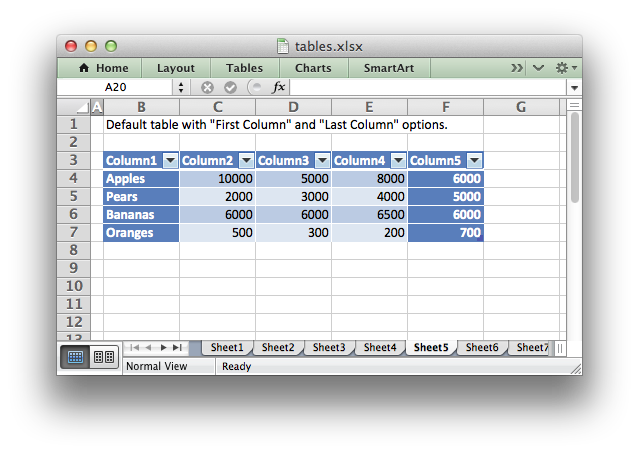## Parameter: last_column

The `last_column` parameter can be used to highlight the last column of the table. The type of highlighting will depend on the `style` of the table. It may be bold text or a different color. It is off by default:

lxw_table_options options = {.first_column = LXW_TRUE, .last_column = LXW_TRUE};

The `last_column` formatting is shown in the image in the previous section above.

## Parameter: style_type and style_type_number

The `style_type` parameter can be used to set the style of the table, in conjunction with the `style_type_number` parameter:

lxw_table_options options = {
.style_type_number = 11,
};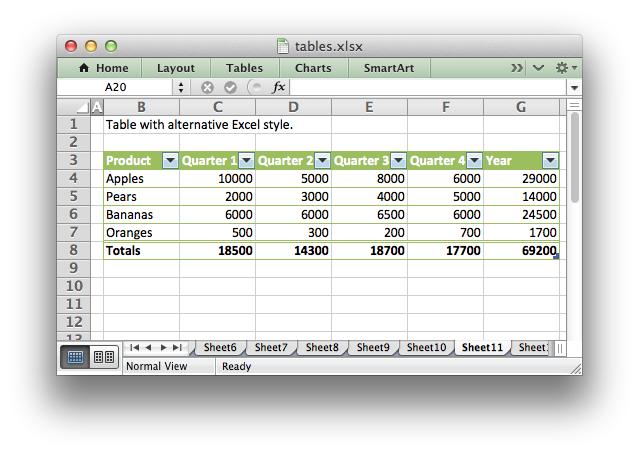There are three types of table style in Excel: Light, Medium and Dark which are represented using the lxw_table_style_type enum values:

Within those ranges there are between 11 and 28 other style types which can be set with `style_type_number` (depending on the style type). Check Excel to find the style that you want. The dialog with the options laid out in numeric order are shown below: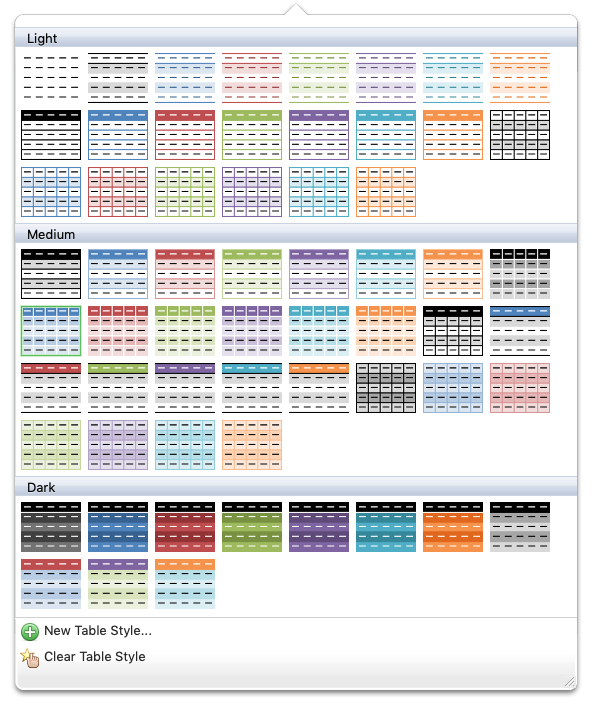The default table style in Excel is 'Table Style Medium 9' (highlighted with a green border in the image above), which is set by default in libxlsxwriter as:

lxw_table_options options = {
.style_type_number = 9,
};

You can also turn the table style off by setting it to Light 0:

lxw_table_options options = {
.style_type_number = 0,
};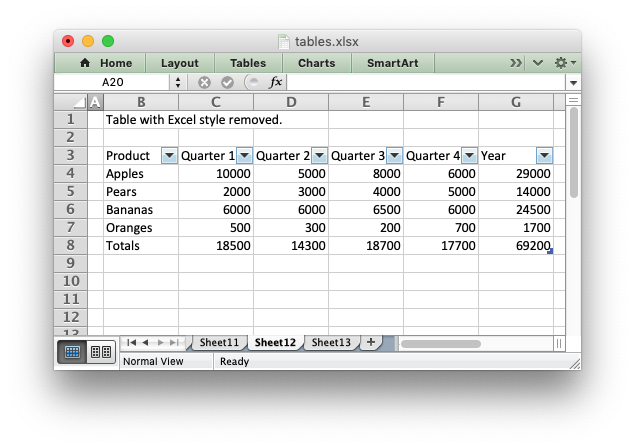## Parameter: name

The `name` parameter is used to set the name of the table. This parameter is optional and by default tables are named `Table1`, `Table2`, etc. in the worksheet order that they are added.

lxw_table_options options = {.name = "Sales"};

If you override the table name you must ensure that it doesn't clash with an existing table name and that it follows Excel's requirements for table names, see the Microsoft Office documentation on Naming an Excel Table.

## Parameter: total_row

The `total_row` parameter can be used to turn on the total row in the last row of a table. It is distinguished from the other rows by a different formatting and also with dropdown `SUBTOTAL` functions: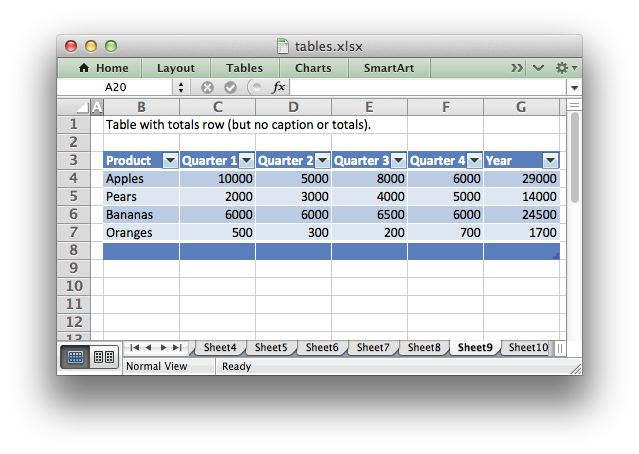The default total row doesn't have any captions or functions. These must by specified via the `columns` parameter below.

## Parameter: columns

The `columns` parameter can be used to set properties for columns within the table.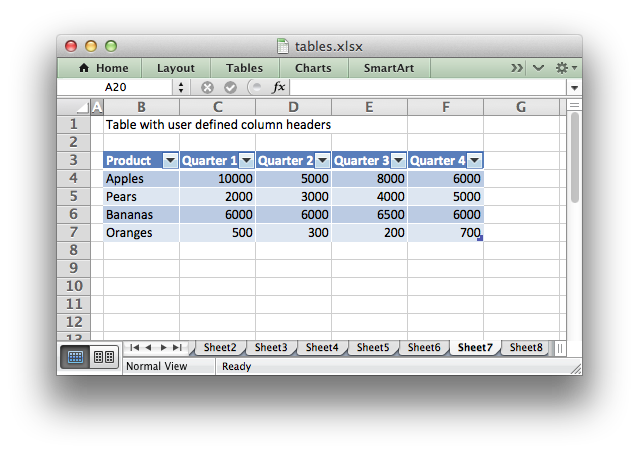The sub-properties of `lxw_table_column` that can be set are:

• `lxw_table_column.header`
• `lxw_table_column.header_format`
• `lxw_table_column.formula`
• `lxw_table_column.total_string`
• `lxw_table_column.total_function`
• `lxw_table_column.total_value`
• `lxw_table_column.format`

The `columns` parameter should be a NULL terminated array of `lxw_table_column` pointers. For example to override the default 'Column n' style table headers:

lxw_table_column col1 = {.header = "Product"};
lxw_table_column col2 = {.header = "Quarter 1"};
lxw_table_column col3 = {.header = "Quarter 2"};
lxw_table_column col4 = {.header = "Quarter 3"};
lxw_table_column col5 = {.header = "Quarter 4"};
lxw_table_column *columns[] = {&col1, &col2, &col3, &col4, &col5, NULL};
lxw_table_options options = {.columns = columns};

If you don't wish to specify properties for a specific column you can pass an empty (but not NULL) struct and the defaults will be applied:

lxw_table_column col1 = {.header = "Product"};
lxw_table_column col2 = {.header = "Quarter 1"};
lxw_table_column col3 = {0}; // Defaults to Column3.
lxw_table_column col4 = {.header = "Quarter 3"};
lxw_table_column col5 = {.header = "Quarter 4"};

Column formulas can by applied using the column `formula` property:

lxw_table_column col1 = {.header = "Product"};
lxw_table_column col2 = {.header = "Quarter 1"};
lxw_table_column col3 = {.header = "Quarter 2"};
lxw_table_column col4 = {.header = "Quarter 3"};
lxw_table_column col5 = {.header = "Quarter 4"};
lxw_table_column col6 = {.header = "Year",
.formula = "=SUM(Table8[@[Quarter 1]:[Quarter 4]])"};
lxw_table_column *columns[] = {&col1, &col2, &col3, &col4, &col5, &col6, NULL};
lxw_table_options options = {.columns = columns};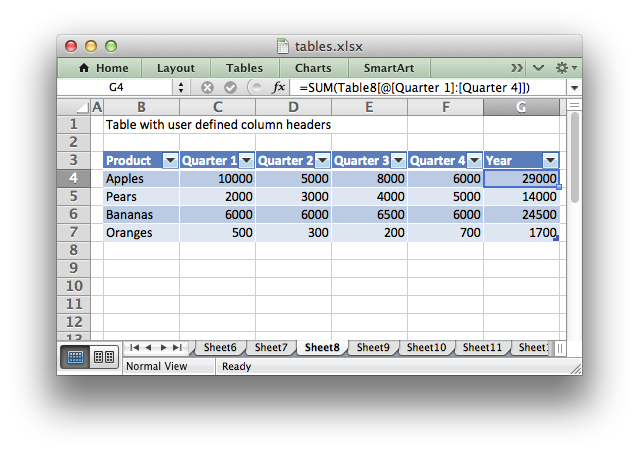The Excel 2007 style `"#This Row"` and Excel 2010 style `"@"` structural references are supported within the formula. However, other Excel 2010 additions to structural references aren't supported and formulas should conform to Excel 2007 style formulas. See the Microsoft documentation on Using structured references with Excel tables for details.

As stated above the `total_row` table parameter turns on the "Total" row in the table but it doesn't populate it with any defaults. Total captions and functions must be specified via the `columns` property and the `total_string` and `total_function` sub properties:

lxw_table_column col1 = {.header = "Product",
.total_string = "Totals"};
lxw_table_column col2 = {.header = "Quarter 1",
.total_function = LXW_TABLE_FUNCTION_SUM};
lxw_table_column col3 = {.header = "Quarter 2",
.total_function = LXW_TABLE_FUNCTION_SUM};
lxw_table_column col4 = {.header = "Quarter 3",
.total_function = LXW_TABLE_FUNCTION_SUM};
lxw_table_column col5 = {.header = "Quarter 4",
.total_function = LXW_TABLE_FUNCTION_SUM};
lxw_table_column col6 = {.header = "Year",
.formula = "=SUM(Table10[@[Quarter 1]:[Quarter 4]])",
.total_function = LXW_TABLE_FUNCTION_SUM};
lxw_table_column *columns[] = {&col1, &col2, &col3, &col4, &col5, &col6, NULL};
lxw_table_options options = {.total_row = LXW_TRUE, .columns = columns};

The supported totals row `SUBTOTAL` functions are defined in lxw_table_total_functions:

User defined functions or formulas aren't supported.

It is also possible to set a calculated value for the `total_function` using the `total_value` sub property. This is only necessary when creating workbooks for applications that cannot calculate the value of formulas automatically. This is similar to setting the `result` property in `worksheet_write_formula_num()`. See also Formula Results.

Formatting can also be applied to columns using the `format` and to the header using `header_format` (although you will also need to add it to the data in the column, see the next section):

lxw_table_column col1 = {.header = "Product",
.total_string = "Totals"};
lxw_table_column col2 = {.header = "Quarter 1",
.total_function = LXW_TABLE_FUNCTION_SUM,
.format = currency_format};
lxw_table_column col3 = {.header = "Quarter 2",
.total_function = LXW_TABLE_FUNCTION_SUM,
.format = currency_format};
lxw_table_column col4 = {.header = "Quarter 3",
.total_function = LXW_TABLE_FUNCTION_SUM,
.format = currency_format};
lxw_table_column col5 = {.header = "Quarter 4",
.total_function = LXW_TABLE_FUNCTION_SUM,
.format = currency_format};
lxw_table_column col6 = {.header = "Year",
.formula = "=SUM(Table13[@[Quarter 1]:[Quarter 4]])",
.total_function = LXW_TABLE_FUNCTION_SUM,
.format = currency_format};
lxw_table_column *columns[] = {&col1, &col2, &col3, &col4, &col5, &col6, NULL};
lxw_table_options options = {.total_row = LXW_TRUE, .columns = columns};Standard libxlsxwriter lxw_format objects are used for this formatting. However, they should be limited to numerical formats for the columns and simple formatting like text wrap for the headers. Overriding other table formatting may produce inconsistent results. You will also need to apply the same format to any data you write to the column in the table, see the next section.

# Adding data to the table

Once you create a worksheet table you will also need to fill in the data in the rows and columns. This is done with the standard worksheet write() functions. For example the data in the examples above was written as follows:

worksheet_write_string(worksheet, 3, 1, "Apples", NULL);
worksheet_write_string(worksheet, 4, 1, "Pears", NULL);
worksheet_write_string(worksheet, 5, 1, "Bananas", NULL);
worksheet_write_string(worksheet, 6, 1, "Oranges", NULL);
worksheet_write_number(worksheet, 3, 2, 10000, format);
worksheet_write_number(worksheet, 4, 2, 2000, format);
worksheet_write_number(worksheet, 5, 2, 6000, format);
worksheet_write_number(worksheet, 6, 2, 500, format);
worksheet_write_number(worksheet, 3, 3, 5000, format);
worksheet_write_number(worksheet, 4, 3, 3000, format);
worksheet_write_number(worksheet, 5, 3, 6000, format);
worksheet_write_number(worksheet, 6, 3, 300, format);
worksheet_write_number(worksheet, 3, 4, 8000, format);
worksheet_write_number(worksheet, 4, 4, 4000, format);
worksheet_write_number(worksheet, 5, 4, 6500, format);
worksheet_write_number(worksheet, 6, 4, 200, format);
worksheet_write_number(worksheet, 3, 5, 6000, format);
worksheet_write_number(worksheet, 4, 5, 5000, format);
worksheet_write_number(worksheet, 5, 5, 6000, format);
worksheet_write_number(worksheet, 6, 5, 700, format);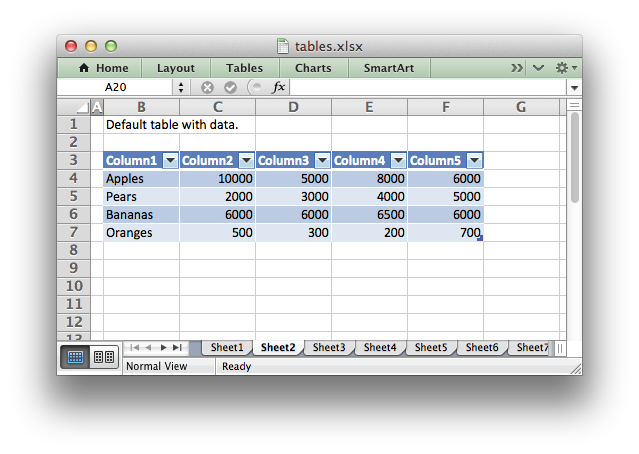# Example

All of the images shown above are taken from tables.c.

lxw_table_options::first_column
uint8_t first_column
Definition: worksheet.h:1523
lxw_table_options::total_row
uint8_t total_row
Definition: worksheet.h:1619
lxw_table_options::style_type
uint8_t style_type
Definition: worksheet.h:1596
lxw_table_options::no_autofilter
uint8_t no_autofilter
Definition: worksheet.h:1477
lxw_table_options::banded_columns
uint8_t banded_columns
Definition: worksheet.h:1507
Definition: worksheet.h:1392
lxw_table_options::no_banded_rows
uint8_t no_banded_rows
Definition: worksheet.h:1492
LXW_TABLE_STYLE_TYPE_MEDIUM
@ LXW_TABLE_STYLE_TYPE_MEDIUM
Definition: worksheet.h:551
RANGE
#define RANGE(range)
Convert an Excel A1:B2 range into a (first_row, first_col, last_row, last_col) sequence.
Definition: utility.h:82
LXW_TRUE
@ LXW_TRUE
Definition: common.h:53
lxw_table_column
Table columns options.
Definition: worksheet.h:1388
lxw_table_options::name
char * name
Definition: worksheet.h:1440
LXW_TABLE_FUNCTION_SUM
@ LXW_TABLE_FUNCTION_SUM
Definition: worksheet.h:587
worksheet_write_string
lxw_error worksheet_write_string(lxw_worksheet *worksheet, lxw_row_t row, lxw_col_t col, const char *string, lxw_format *format)
Write a string to a worksheet cell.
lxw_error worksheet_add_table(lxw_worksheet *worksheet, lxw_row_t first_row, lxw_col_t first_col, lxw_row_t last_row, lxw_col_t last_col, lxw_table_options *options)
Add an Excel table to a worksheet.
worksheet_write_number
lxw_error worksheet_write_number(lxw_worksheet *worksheet, lxw_row_t row, lxw_col_t col, double number, lxw_format *format)
Write a number to a worksheet cell.
lxw_table_options
Worksheet table options.
Definition: worksheet.h:1421
LXW_TABLE_STYLE_TYPE_LIGHT
@ LXW_TABLE_STYLE_TYPE_LIGHT
Definition: worksheet.h:548
lxw_table_options::columns
lxw_table_column ** columns
Definition: worksheet.h:1626